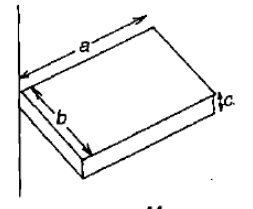Select Question Set:# The radius of gyration of a uniform rod of length L about an axis passing through its centre of mass is 1. $\frac{L}{2\sqrt{3}}$ 2. $\frac{{L}^{2}}{12}$ 3. $\frac{L}{\sqrt{3}}$ 4. $\frac{L}{\sqrt{2}}$Subtopic:  Moment of Inertia |
73%
From NCERT
To view explanation, please take trial in the course.
NEET 2023 - Target Batch - Aryan Raj Singh
Hints
To view explanation, please take trial in the course.
NEET 2023 - Target Batch - Aryan Raj Singh

Two uniform, thin, identical rods, each of mass M and length l are joined together to form a cross. What will be the moment of inertia of the cross about an axis passing through the point at which the two rods are joined and are perpendicular to the plane of the cross?

1. $\frac{M{l}^{2}}{12}$

2. $\frac{M{l}^{2}}{6}$

3. $\frac{M{l}^{2}}{4}$

4. $\frac{M{l}^{2}}{3}$Subtopic:  Moment of Inertia |
77%
From NCERT
To view explanation, please take trial in the course.
NEET 2023 - Target Batch - Aryan Raj Singh
Hints
To view explanation, please take trial in the course.
NEET 2023 - Target Batch - Aryan Raj Singh

If the linear momentum is increased by 50%, the kinetic energy will increase by

1.  50%

2.  100%

3.  125%

4.  25%Subtopic:  Linear Momentum |
63%
From NCERT
To view explanation, please take trial in the course.
NEET 2023 - Target Batch - Aryan Raj Singh
Hints
To view explanation, please take trial in the course.
NEET 2023 - Target Batch - Aryan Raj Singh

If the kinetic energy of a body increases by 0.1%, the percent increase of its momentum will be

1.  0.05%

2.  0.1%

3.  1.0%

4.  10%Subtopic:  Linear Momentum |
71%
From NCERT
To view explanation, please take trial in the course.
NEET 2023 - Target Batch - Aryan Raj Singh
Hints
To view explanation, please take trial in the course.
NEET 2023 - Target Batch - Aryan Raj Singh

A solid cylinder and a hollow cylinder, both of the same mass and same external diameter are released from the same height at the same time on an inclined plane. Both roll down without slipping. Which one will reach the bottom first?

1.  Both together only when the angle of inclination of the plane is ${45}^{0}$

2.  Both together

3.  Hollow cylinder

4.  Solid cylinderSubtopic:  Rolling Motion (OLD NCERT) |
63%
From NCERT
To view explanation, please take trial in the course.
NEET 2023 - Target Batch - Aryan Raj Singh
Hints
To view explanation, please take trial in the course.
NEET 2023 - Target Batch - Aryan Raj Singh

A man is sitting on a rotating table with his arms stretched outwards.  When he suddenly folds his arms inside, then

1. his angular velocity will decrease

2. his angular velocity remains constant

3. his moment of inertia decreases

4. angular momentum increasesSubtopic:  Moment of Inertia |
67%
From NCERT
To view explanation, please take trial in the course.
NEET 2023 - Target Batch - Aryan Raj Singh
Hints
To view explanation, please take trial in the course.
NEET 2023 - Target Batch - Aryan Raj Singh

A body of mass $$M$$ and radius $$R$$ is rolling horizontally without slipping with speed $$v.$$ It then rolls up a hill to a maximum height $$h.$$ If $$h=\frac{5v^{2}}{6g},$$ what is the moment of inertia of the body?
1. $$\frac{MR^{2}}{2}$$
2. $$\frac{2MR^{2}}{3}$$
3. $$\frac{3MR^{2}}{4}$$
4. $$\frac{2MR^{2}}{5}$$Subtopic:  Rolling Motion (OLD NCERT) |
75%
From NCERT
To view explanation, please take trial in the course.
NEET 2023 - Target Batch - Aryan Raj Singh
Hints
To view explanation, please take trial in the course.
NEET 2023 - Target Batch - Aryan Raj Singh

One circular ring and one circular disc both having the same mass and radius. The ratio of their moments of inertia about the axes passing through their centres and perpendicular to planes will be

1. 1:1

2. 2:1

3. 1:2

4. 4:1Subtopic:  Moment of Inertia |
85%
From NCERT
To view explanation, please take trial in the course.
NEET 2023 - Target Batch - Aryan Raj Singh
Hints
To view explanation, please take trial in the course.
NEET 2023 - Target Batch - Aryan Raj Singh

A wheel of radius R rolls on the ground with a uniform velocity v. The velocity of topmost point relative to the bottommost point is

1. v

2. 2v

3. v/2

4. zeroSubtopic:  Rolling Motion (OLD NCERT) |
71%
From NCERT
To view explanation, please take trial in the course.
NEET 2023 - Target Batch - Aryan Raj Singh
Hints
To view explanation, please take trial in the course.
NEET 2023 - Target Batch - Aryan Raj Singh

The figure shows a uniform solid block of mass M and edge lengths a, b and c. Its M.O.I. about an axis through one edge and perpendicular (as shown) to the large face of the block is1. $\frac{M}{3}\left({a}^{2}+{b}^{2}\right)$

2. $\frac{M}{4}\left({a}^{2}+{b}^{2}\right)$

3. $\frac{7M}{12}\left({a}^{2}+{b}^{2}\right)$

4. $\frac{M}{12}\left({a}^{2}+{b}^{2}\right)$Subtopic:  Moment of Inertia |
51%
To view explanation, please take trial in the course.
NEET 2023 - Target Batch - Aryan Raj Singh
Hints

Select Question Set: